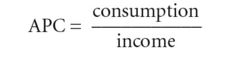# average propensity to consume

Also found in: Acronyms, Wikipedia.

## Average Propensity to Consume

The amount of money a person spends as a percentage of total income. For example, if one makes \$50,000 and spends \$40,000, the average propensity to consume is 80%. Countries with a high average propensity to consume generally have a lower unemployment rate because the demand to buy things creates jobs. However, it may be more susceptible to recession as people save very little. It should not be confused with the marginal propensity to consume.

## average propensity to consume (APC)

the fraction of a given level of NATIONAL INCOME that is spent on consumption:Alternatively, consumption can be expressed as a proportion of DISPOSABLE INCOME. See CONSUMPTION EXPENDITURE, PROPENSITY TO CONSUME, MARGINAL PROPENSITY TO CONSUME.

Mentioned in ?
References in periodicals archive ?
Some of the findings in this paper are that not only is the equation of exchange derived from a utility maximizing model and that velocity is constant when interest rates are fixed, but it is demonstrated that net wealth is positively related to the average propensity to consume, an outcome that supports the positive relationship between wealth and consumption.
This result confirms that consumption is proportional to income and net-wealth; that the average propensity to consume (APC) is decreasing in income (equation 12), but increasing in net-wealth, with the marginal propensity to consume (MPC) being constant (equation 13):
The former decreases the average propensity to consume, freeing up resources for the government.
This important empirical finding makes the old "consumption puzzle" more intriguing because it shows that the average propensity to consume decreases not only with current income, but also with lifetime income.
An important component of Keynes' general theory (1936) is the Keynesian consumption function, which implies that the average propensity to consume decreases with income.
It also is of grave concern that the average propensity to consume has begun to decline.
The additional national saving after a policy change is estimated by computing each generation's implied change in total resources times its age-specific average propensity to consume.
t-1] = Lagged average propensity to consume used as a proxy for wealth variable.
It may be pointed out that instead of consumption expenditure we use average propensity to consume as an endogenous variable because we believe that advertising intensity will influence the propensity to consume more than the consumption level itself.
MATHEMATICAL EXPRESSION NOT REPRODUCIBLE IN ASCII], the average propensity to consume permanent income equals the marginal propensity to consume permanent income.
If the permanent-income theory is entirely correct, then the marginal propensity to consume, when computed from the nationality grouping, should equal the average propensity to consume.

Site: Follow: Share:
Open / Close